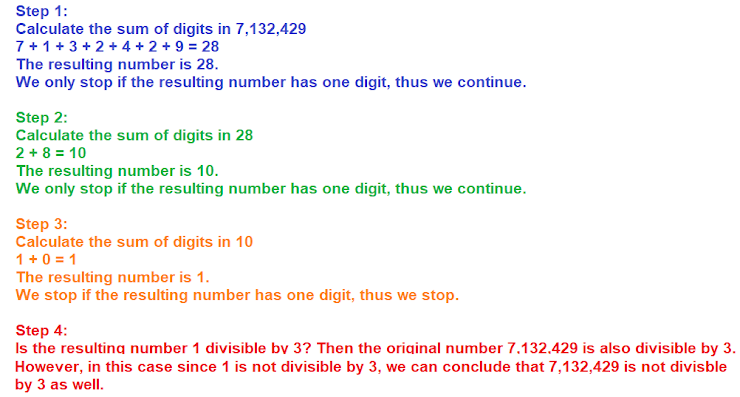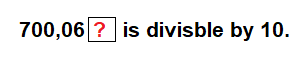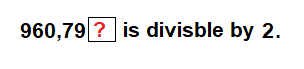Middle School Math, Warm-Up #5
Divisibility
Divisibility is an important skill for learning factoring, relationships between prime numbers, and more.
A number is divisible by 3 if
1 point
The following method decides if 7,132,429 is divisible by 3? Is this method correct?
1 pointType a digit that makes the following statement true.
1 pointType any digit that makes the following statement true.
1 pointChoose a digit to replace the question mark so that this statement is true: 3,896,78? is divisible by 2.
1 point
Is this statement correct? "A number is divisible by 5 only if it ends with either 0 or 5."
1 point
Is 384,752 divisible by 5?
1 point
Select all the digits that can replace the question mark to make this statement true: 18,673,92? is divisible by 3.
1 point